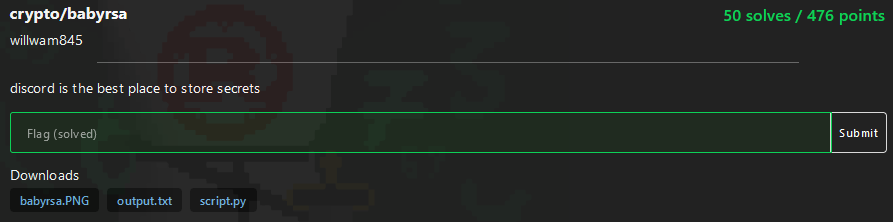# corCTF 2021 - babyrsa

Cryptography – 476 pts (50 solves) – Chall author: willwam845

Somebody leaked a screenshot of a Discord chat with the author’s RSA modulus primes! Well, partially… Turns out we are missing some of the digits, but not enough to stop us from recovering his private exponent and steal his flag. >:)

Check out write-ups by my teammates on K3RN3L4RMY.com

## ExplorationWe are presented with the following leak from the author’s Discord chat and its transcription.Transcription of image:
735426165606478775655440367887380252029393814251587377215443983568517874011835161632
289108065126883603562904941748653607836358267359664041064708762154474786168204628181
9145476916585122917284319282272004045859138239853037072761
108294440701045353595867242719660522374526250640690193563048263854806748525172379331
341078269246532299656864881223
679098724593514422867704492870375465007225641192338424726642090768164214390632598250
39563231146143146482074105407

(n, p, q)


The source code output also displays the public modulus along with the public exponent and the ciphertext.

n = 73542616560647877565544036788738025202939381425158737721544398356851787401183516163221837013929559568993844046804187977705376289108065126883603562904941748653607836358267359664041064708762154474786168204628181667371305788303624396903323216279110685399145476916585122917284319282272004045859138239853037072761
e = 65537
ct = 2657054880167593054409755786316190176139048369036893368834913798649283717358246457720021168590230987384201961744917278479195838455294205306264398417522071058105245210332964380113841646083317786151272874874267948107036095666198197073147087762030842808562672646078089825632314457231611278451324232095496184838


By comparing the full public modulus with the transcipt of the modulus in the image we see that there are two gaps of 41 missing digits in it. This means we only have partial knowledge of ‘p’ and ‘q’, but perhaps it is enough to infer their full values such that we can crack this flag.

## Exploitation

Our first step is to write ‘p’ and ‘q’ out in terms of the unknown digit blocks, which I will call ‘x’ and ‘y’:

$p = A 10^{71} + x 10^{30} + B$

and

$q = C 10^{70} + y 10^{29} + D$

where

A = 108294440701045353595867242719660522374526250640690193563048263854806748525172379331
x = ?
B = 341078269246532299656864881223

C = 679098724593514422867704492870375465007225641192338424726642090768164214390632598250
y = ?
D = 39563231146143146482074105407


However, we only have to solve for one prime as we can find the other through dividing the modulus by our recovered prime. So let’s focus on ‘p’ and create a univariate (only one variable, ‘x’) polynomial using Sage. Note that we need to make the polynomial monic, coefficient of ‘x’ is 1, for Sage to help us successfully.

# Set up a univariate polynomial
P.<x> = PolynomialRing(Integers(n))
p = A*10**71 + x*10**30 + B
# Make it monic
p *= inverse_mod(10**30,n)
print(p)

x + 12982249919280027255436123729204655331755401707242095380530980282681281795515879103774877710047957413839981165090649359723107955263470400087152178392809127231166537983078813053595286483448018264573858966385470051532465449907432854932644567644490432882163835408920633304531497120952928599612084062010297989201


Alright, now it’s time for Sage to do it’s magic!

p.small_roots()

[]


Oh… nothing, eerhm. So are we doomed? No, there is something we can do, and that is help Sage a little bit by providing an absolute bound for x (‘X = 10**41’) and a smaller beta value than 1 (‘beta = 1/10’).

p.small_roots(X=10**41,beta=1/10)




Teamwork makes the dream work! Now let’s use our solution to find ‘p’ and crack the flag!

psol = p.small_roots(X=10**41, beta=1/10)
P = int(A*10**71 + psol*10**30 + B)

assert n % P == 0
Q = n//P

print('P =',P)
print('Q =',Q)

f = (P-1)*(Q-1)
d = inverse(e,f)

pt = pow(ct,d,n)
print(long_to_bytes(pt))

P = 10829444070104535359586724271966052237452625064069019356304826385480674852517237933153064479807396839988036432397395969825028341078269246532299656864881223
Q = 6790987245935144228677044928703754650072256411923384247266420907681642143906325982502924686164657029534309292920660089520491839563231146143146482074105407
b'corctf{1_w4s_f0rc3d_t0_wr1t3_ch4ll5_4nd_1_h4d_n0_g00d_1d345_pl5_n0_bully_;-;}'


Ta-da!

corctf{1_w4s_f0rc3d_t0_wr1t3_ch4ll5_4nd_1_h4d_n0_g00d_1d345_pl5_n0_bully_;-;}